Anúncio

# COOPERATIVE QUIZ.pptx

26 de Mar de 2023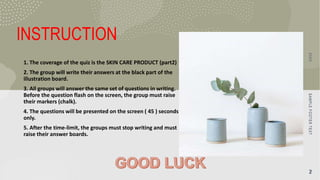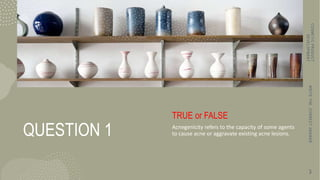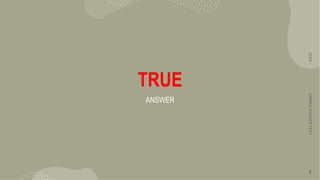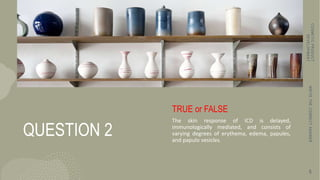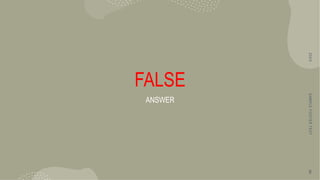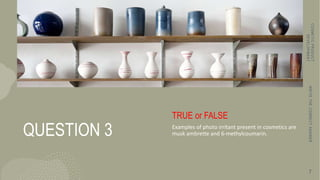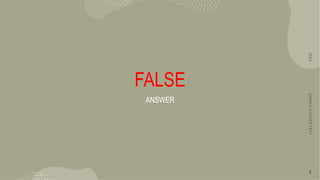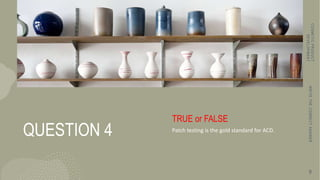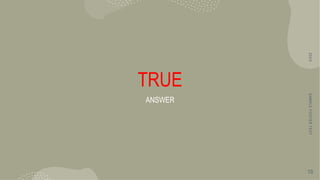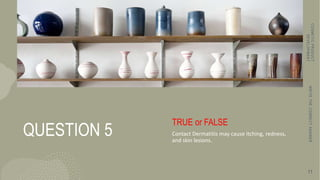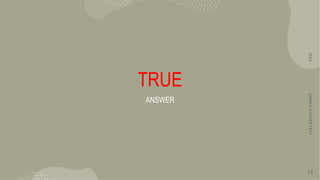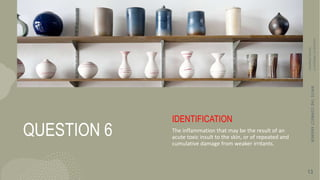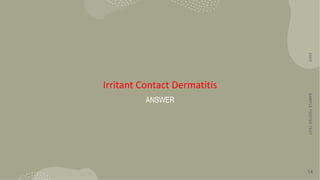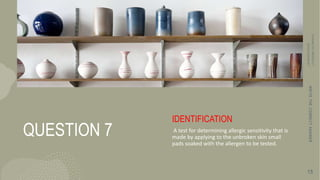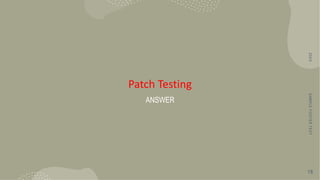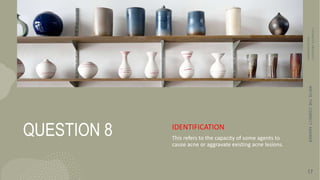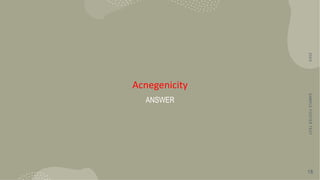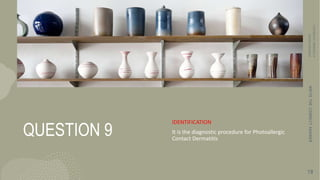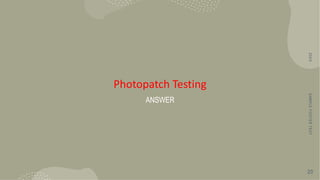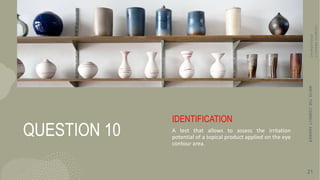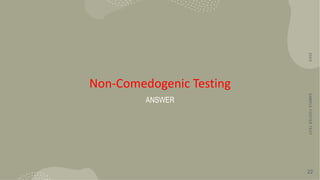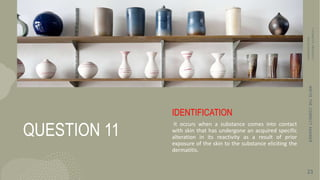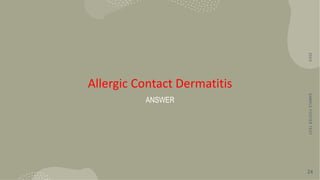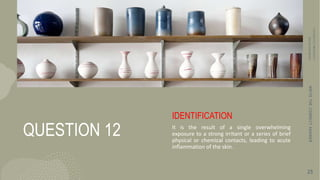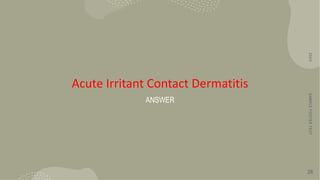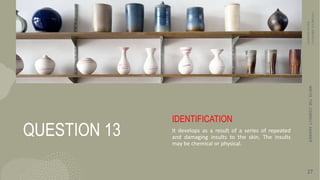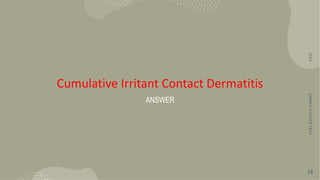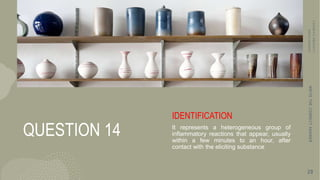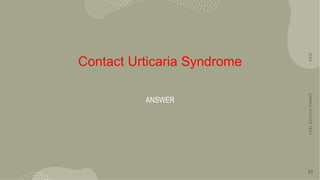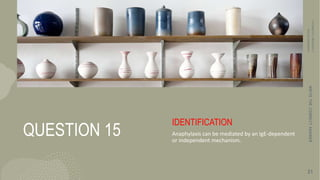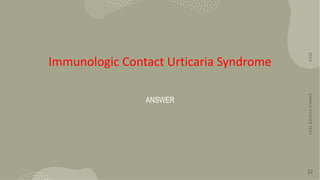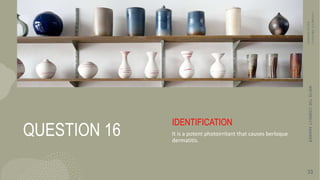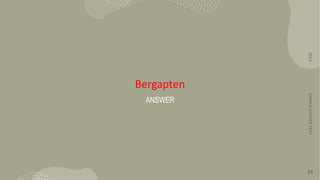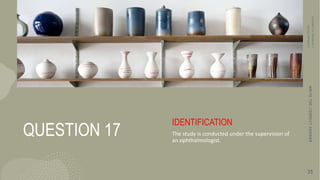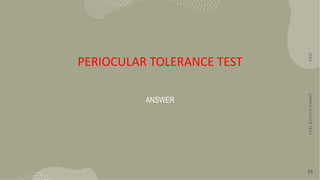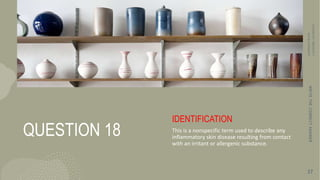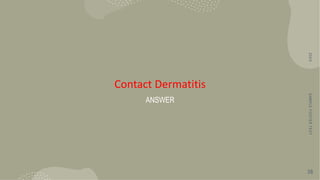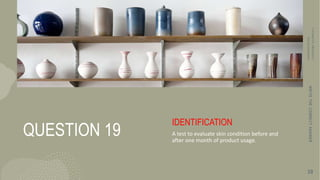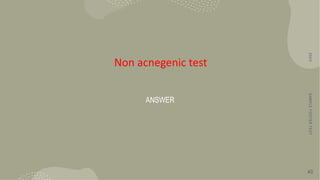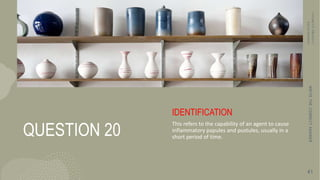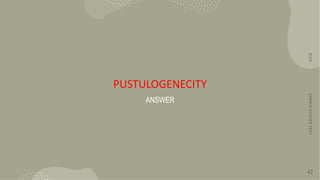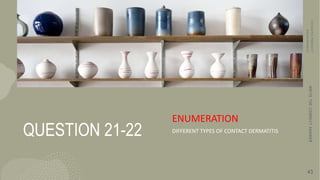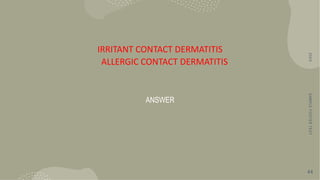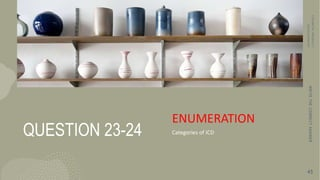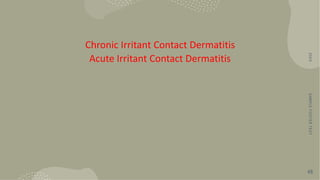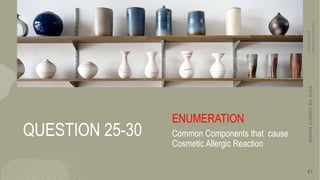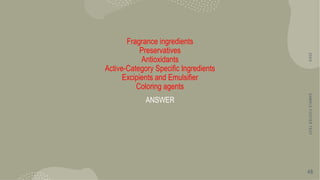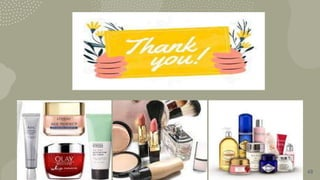1 de 49
Anúncio

### COOPERATIVE QUIZ.pptx

1. COOPERATIVE QUIZ COSMETIC DEVELOPMENT
2. S A M P L E F O O T E R T E X T 2 INSTRUCTION 1. The coverage of the quiz is the SKIN CARE PRODUCT (part2) 2. The group will write their answers at the black part of the illustration board. 3. All groups will answer the same set of questions in writing. Before the question flash on the screen, the group must raise their markers (chalk). 4. The questions will be presented on the screen ( 45 ) seconds only. 5. After the time-limit, the groups must stop writing and must raise their answer boards. 2 0 X X S A M P L E F O O T E R T E X T 2
3. QUESTION 1 TRUE or FALSE Acnegenicity refers to the capacity of some agents to cause acne or aggravate existing acne lesions. C O S M E T I C P R O D U C T D E V E L O P M E N T W R I T E T H E C O R R E C T A N S W E R 3
4. TRUE ANSWER 2 0 X X S A M P L E F O O T E R T E X T 4
5. QUESTION 2 TRUE or FALSE The skin response of ICD is delayed, immunologically mediated, and consists of varying degrees of erythema, edema, papules, and papulo vesicles. C O S M E T I C P R O D U C T D E V E L O P M E N T W R I T E T H E C O R R E C T A N S W E R 5
6. FALSE ANSWER 2 0 X X S A M P L E F O O T E R T E X T 6
7. QUESTION 3 TRUE or FALSE Examples of photo irritant present in cosmetics are musk ambrette and 6-methylcoumarin. C O S M E T I C P R O D U C T D E V E L O P M E N T W R I T E T H E C O R R E C T A N S W E R 7
8. FALSE ANSWER 2 0 X X S A M P L E F O O T E R T E X T 8
9. QUESTION 4 TRUE or FALSE Patch testing is the gold standard for ACD. C O S M E T I C P R O D U C T D E V E L O P M E N T W R I T E T H E C O R R E C T A N S W E R 9
10. TRUE ANSWER 2 0 X X S A M P L E F O O T E R T E X T 10
11. QUESTION 5 TRUE or FALSE Contact Dermatitis may cause itching, redness, and skin lesions. C O S M E T I C P R O D U C T D E V E L O P M E N T W R I T E T H E C O R R E C T A N S W E R 11
12. TRUE ANSWER 2 0 X X S A M P L E F O O T E R T E X T 12
13. QUESTION 6 IDENTIFICATION The inflammation that may be the result of an acute toxic insult to the skin, or of repeated and cumulative damage from weaker irritants. W R I T E T H E C O R R E C T A N S W E R 13
14. Irritant Contact Dermatitis ANSWER 2 0 X X S A M P L E F O O T E R T E X T 14
15. QUESTION 7 IDENTIFICATION A test for determining allergic sensitivity that is made by applying to the unbroken skin small pads soaked with the allergen to be tested. W R I T E T H E C O R R E C T A N S W E R 15
16. Patch Testing ANSWER 2 0 X X S A M P L E F O O T E R T E X T 16
17. QUESTION 8 IDENTIFICATION This refers to the capacity of some agents to cause acne or aggravate existing acne lesions. W R I T E T H E C O R R E C T A N S W E R 17
18. Acnegenicity ANSWER 2 0 X X S A M P L E F O O T E R T E X T 18
19. QUESTION 9 IDENTIFICATION It is the diagnostic procedure for Photoallergic Contact Dermatitis W R I T E T H E C O R R E C T A N S W E R 19
20. Photopatch Testing ANSWER 2 0 X X S A M P L E F O O T E R T E X T 20
21. QUESTION 10 IDENTIFICATION A test that allows to assess the irritation potential of a topical product applied on the eye contour area. W R I T E T H E C O R R E C T A N S W E R 21
22. Non-Comedogenic Testing ANSWER 2 0 X X S A M P L E F O O T E R T E X T 22
23. QUESTION 11 IDENTIFICATION It occurs when a substance comes into contact with skin that has undergone an acquired specific alteration in its reactivity as a result of prior exposure of the skin to the substance eliciting the dermatitis. W R I T E T H E C O R R E C T A N S W E R 23
24. Allergic Contact Dermatitis ANSWER 2 0 X X S A M P L E F O O T E R T E X T 24
25. QUESTION 12 IDENTIFICATION It is the result of a single overwhelming exposure to a strong irritant or a series of brief physical or chemical contacts, leading to acute inflammation of the skin. W R I T E T H E C O R R E C T A N S W E R 25
26. Acute Irritant Contact Dermatitis ANSWER 2 0 X X S A M P L E F O O T E R T E X T 26
27. QUESTION 13 IDENTIFICATION It develops as a result of a series of repeated and damaging insults to the skin. The insults may be chemical or physical. W R I T E T H E C O R R E C T A N S W E R 27
28. Cumulative Irritant Contact Dermatitis ANSWER 2 0 X X S A M P L E F O O T E R T E X T 28
29. QUESTION 14 IDENTIFICATION It represents a heterogeneous group of inflammatory reactions that appear, usually within a few minutes to an hour, after contact with the eliciting substance W R I T E T H E C O R R E C T A N S W E R 29
30. Contact Urticaria Syndrome ANSWER 2 0 X X S A M P L E F O O T E R T E X T 30
31. QUESTION 15 IDENTIFICATION Anaphylaxis can be mediated by an IgE-dependent or independent mechanism. W R I T E T H E C O R R E C T A N S W E R 31
32. Immunologic Contact Urticaria Syndrome ANSWER 2 0 X X S A M P L E F O O T E R T E X T 32
33. QUESTION 16 IDENTIFICATION It is a potent photoirritant that causes berloque dermatitis. W R I T E T H E C O R R E C T A N S W E R 33
34. Bergapten ANSWER 2 0 X X S A M P L E F O O T E R T E X T 34
35. QUESTION 17 IDENTIFICATION The study is conducted under the supervision of an ophthalmologist. W R I T E T H E C O R R E C T A N S W E R 35
36. PERIOCULAR TOLERANCE TEST ANSWER 2 0 X X S A M P L E F O O T E R T E X T 36
37. QUESTION 18 IDENTIFICATION This is a nonspecific term used to describe any inflammatory skin disease resulting from contact with an irritant or allergenic substance. W R I T E T H E C O R R E C T A N S W E R 37
38. Contact Dermatitis ANSWER 2 0 X X S A M P L E F O O T E R T E X T 38
39. QUESTION 19 IDENTIFICATION A test to evaluate skin condition before and after one month of product usage. W R I T E T H E C O R R E C T A N S W E R 39
40. Non acnegenic test ANSWER 2 0 X X S A M P L E F O O T E R T E X T 40
41. QUESTION 20 IDENTIFICATION This refers to the capability of an agent to cause inflammatory papules and pustules, usually in a short period of time. W R I T E T H E C O R R E C T A N S W E R 41
42. PUSTULOGENECITY ANSWER 2 0 X X S A M P L E F O O T E R T E X T 42
43. QUESTION 21-22 ENUMERATION DIFFERENT TYPES OF CONTACT DERMATITIS W R I T E T H E C O R R E C T A N S W E R 43
44. IRRITANT CONTACT DERMATITIS ALLERGIC CONTACT DERMATITIS ANSWER 2 0 X X S A M P L E F O O T E R T E X T 44
45. QUESTION 23-24 ENUMERATION Categories of ICD W R I T E T H E C O R R E C T A N S W E R 45
46. Chronic Irritant Contact Dermatitis Acute Irritant Contact Dermatitis 2 0 X X S A M P L E F O O T E R T E X T 46
47. QUESTION 25-30 ENUMERATION Common Components that cause Cosmetic Allergic Reaction W R I T E T H E C O R R E C T A N S W E R 47
48. Fragrance ingredients Preservatives Antioxidants Active-Category Specific Ingredients Excipients and Emulsifier Coloring agents ANSWER 2 0 X X S A M P L E F O O T E R T E X T 48
49. 49
Anúncio